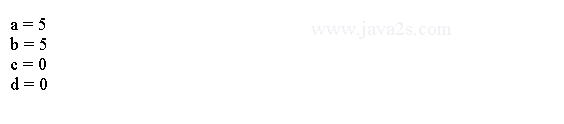# Java Tutorial - Java If

Java if statement is used to execute a block of code based on a condition.

## Java If Statement

The following the simplest form of Java if statement:

```if(condition)
statement;
```

`condition` is a boolean expression. If `condition` is `true`, then the `statement` is executed.

If `condition` is `false`, then the `statement` is bypassed.

The following code outputs a message based on the value of an integer. It uses the if statement to do the check.

```public class Main {
/*from   w w w  .j av a 2s . c om*/
public static void main(String args[]) {
int num = 99;
if (num < 100) {
System.out.println("num is less than 100");

}
}
}
```

The output generated by this program is shown here: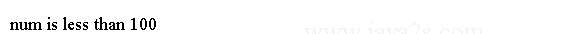## Example

If statement is often used to to compare two variables. The following code defines two variables, `x` and `y`, the it uses the if statement to compare them and prints out messages.

```public class Main {
//  w  w  w  .  j  ava 2s . c  om
public static void main(String args[]) {
int x, y;

x = 10;
y = 20;

if (x < y){
System.out.println("x is less than y");
}

x = x * 2;
if (x == y){
System.out.println("x now equal to y");
}

x = x * 2;
if (x > y){
System.out.println("x now greater than y");
}

if (x == y){
System.out.println("===");
}
}
}
```

The output generated by this program is shown here:## Example 2

We can also use a boolean value to control the if statement. The value of a `boolean` variable is sufficient, by itself, to control the if statement.

```public class Main {
public static void main(String args[]) {
boolean b;//from   www .ja  v  a  2s.  c om
b = false;
if (b) {
System.out.println("This is executed.");
} else {
System.out.println("This is NOT executed.");
}

}
}
```

There is no need to write an `if` statement like this:

```if(b == true) ...
```

The output generated by this program is shown here: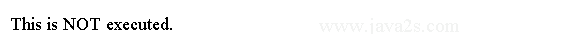## Java if else Statement

The `if` statement is a conditional branch statement. We can add else statement to the if statement.

Here is the general form of the `if-else` statement:

```if (condition)
statement1;
else
statement2;
```

The `else` clause is optional. Each statement may be a single statement or a compound statement enclosed in curly braces (a block). Only one statement can appear directly after the `if` or the `else`. To include more statements, you'll need to create a block, as in this fragment.

The following example shows how to use Java `if else` statement.

```
public class Main {
public static void main(String[] argv) {
int i = 1;//from   w w w  .  j  a v a 2  s.  c o m

if (i > 0) {
System.out.println("Here");
i -= 1;

} else
System.out.println("There");
}
}
]]>
```

The output: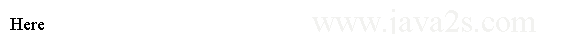It is good to include the curly braces when using the `if` statement, even when there is only one statement in each clause.

## Java if else ladder statement

The if else ladder statement is used to work on multiple conditions.

The if-else-if Ladder looks like this:

```if(condition)
statement;
else if(condition)
statement;
else if(condition)
statement;
.
.
else
statement;
```

Here is a program that uses an `if-else-if` ladder.

```
public class Main {
public static void main(String args[]) {
int month = 4;
String value;/*  ww  w  .j  a va  2s.  c om*/
if (month == 1 )
value = "A";
else if (month == 2)
value = "B";
else if (month == 3)
value = "C";
else if (month == 4)
value = "D";
else
value = "Error";

System.out.println("value = " + value);
}
}
```

Here is the output produced by the program: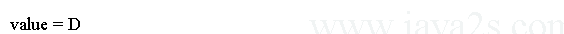## Java nested if statement

A nested `if` is an `if` statement inside another another `if` statement or `else`.

The following code uses a nested if statement to compare values.

```
public class Main {
public static void main(String[] argv) {
int i = 10;/*from w ww .  java  2s .  c o m*/
int j = 4;
int k = 200;
int a = 3;
int b = 5;
int c = 0;
int d =0;
if (i == 10) {
if (j < 20){
a = b;
}
if (k > 100){
c = d;
}
else{
a = c;
}
} else{
a = d;
}
System.out.println("a = " + a);
System.out.println("b = " + b);
System.out.println("c = " + c);
System.out.println("d = " + d);

}
}
```

The output: